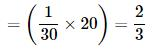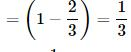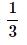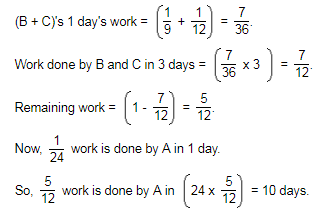Courses

# Time And Work MCQ 2

## 20 Questions MCQ Test Quantitative Techniques for CLAT | Time And Work MCQ 2

Description
This mock test of Time And Work MCQ 2 for Quant helps you for every Quant entrance exam. This contains 20 Multiple Choice Questions for Quant Time And Work MCQ 2 (mcq) to study with solutions a complete question bank. The solved questions answers in this Time And Work MCQ 2 quiz give you a good mix of easy questions and tough questions. Quant students definitely take this Time And Work MCQ 2 exercise for a better result in the exam. You can find other Time And Work MCQ 2 extra questions, long questions & short questions for Quant on EduRev as well by searching above.
QUESTION: 1

Solution:
QUESTION: 2

Solution:
QUESTION: 3

### A, B, and C can do a piece of work in 24 days. A and B can do it in 40 days, B and C can do it in 30 days. How many days will A and C take to finish work?

Solution:
QUESTION: 4

A and B can do a piece of work in 4 days, B and C in 6 days and A, B and C all together in 3 days, what time will A and C take to do it?

Solution:
QUESTION: 5

A and B can together finish a work 30 days. They worked together for 20 days and then B left. After another 20 days, A finished the remaining work. In how many days A alone can finish the work?

Solution:

(A + B)'s 20day's workRemaining workNow,work is done by A in 20 days

∴ The whole work will be done by A in (20×3) = 60days

QUESTION: 6

A and B can do a work in 8 days B and C in 12 days, C and A in 16 days. All worked for 2 days. How many days willA take to finish the work.

Solution:
QUESTION: 7

A can do a piece of work in 12 hours and with the help of his son, he is able to do it in 8 hours. How many hours will the son alone take to finish the work?

Solution:
QUESTION: 8

A pipe can fill a tank in y hours and another can empty it in x hours. They can together fill it in ………(x > y).

Solution:
QUESTION: 9

A tank is filled by a pipeA in 32 min. and pipe B in 36 min. When filled, it can be emptied by pipe C in 20 minute. If all the three pipes are opened simultaneously 1/3rd of tank will be filled in

Solution:
QUESTION: 10

Two pipes x and y can fill a cistern in 24 minute and 32 min. res. If both the pipes are opened together, then after how much time should ybe closed so that tank is full in 18 minutes.

Solution:
QUESTION: 11

Mahesh and Umesh can complete a work in 10 days and 15 days respectively. Umesh starts the work and after 5 days Mahesh also joins him. In all, the work would be completed in

Solution:
QUESTION: 12

Twelve men can complete a work in 8 days. Three days after they started the work, 3 more men joined them. In how many days will all of them together complete the remaining work:

Solution:
QUESTION: 13

The rates of working of A and B are in the ratio 3 : 4. The number of days taken by them, to finish the work are in the ratio:

Solution:
QUESTION: 14

Sunil completes a work in 4 days whereas Hrithik completes the work in 6 days. Ramesh work 1 ½ times as fast as Sunil. How many days it will take for the three together the complete the work?

Solution:
QUESTION: 15

A can do a piece of work in 10 days and B can do the same piece of work in 20 days. They start the work together but after 5 days, A leaves off. B will do the remaining piece of work in :

Solution:
QUESTION: 16

A and B can together finish a work in 30 days. They worked for it for 20 days and then B left. The remaining work done by A alone in 20 more days. A alone can finish the work in

Solution:
QUESTION: 17

A can complete a job in 9 days, B in 10 days and C in 15 days. B and C start the work and are forced to leave after 2 days. The time taken to complete the remaining work by A is

Solution: Correct Option is (C)QUESTION: 18

A, B and C together earn Rs. 150 per day while A & C together earn Rs. 94 and B and C together earn Rs. 76. The daily earning of C is:

Solution:
QUESTION: 19

A is twice as good a workman as B and together they finish a piece of work in 14 days. A alone can finish the work in

Solution:
QUESTION: 20

A is thrice as good as workman as B and takes 10 days less to do a piece of work than B takes. B can do the work in

Solution: